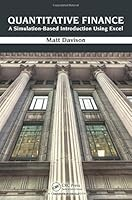# Quantitative Finance: A Simulation-Based Introduction Using Excel2 Reviews
2014-05-08
532 pages

## Book Description

Teach Your Students How to Become Successful Working Quants

Quantitative Finance: A Simulation-Based Introduction Using Excel provides an introduction to financial mathematics for students in applied mathematics, financial engineering, actuarial science, and business . The text not only enables students to practice with the basic techniques of financial mathematics, but it also helps them gain significant intuition about what the techniques mean, how they work, and what happens when they stop working.

After introducing , return, decision making under uncertainty, and traditional discounted cash flow project analysis, the book covers mortgages, bonds, and annuities using a blend of Excel simulation and difference equation or algebraic formalism. It then looks at how interest rate markets work and how to model bond prices before addressing mean variance portfolio optimization, the capital asset pricing model, options, and value at (VaR). The author next focuses on binomial model tools for pricing options and the analysis of discrete random walks. He also introduces stochastic in a nonrigorous way and explains how to simulate geometric Brownian motion. The text proceeds to thoroughly discuss options pricing, mostly in continuous time. It concludes with chapters on stochastic of the yield curve and incomplete markets using simple discrete .

Accessible to students with a relatively modest level of mathematical background, this book will guide your students in becoming successful quants. It uses both hand calculations and Excel spreadsheets to analyze plenty of examples from simple bond portfolios. The spreadsheets are available on the book’s CRC Press web page.

Chapter 1 - Introduction
Chapter 2 - Intuition about Uncertainty and Risk
Chapter 3 - The Classical Approach to Decision Making under Uncertainty
Chapter 4 - Valuing Investment Opportunities: The Discounted Cash Flow Method
Chapter 5 - Repaying Loans over Time
Chapter 6 - Bond Pricing with Default: Using Simulations
Chapter 7 - Bond Pricing with Default: Using Difference Equations
Chapter 8 - Difference Equations for Life Annuities
Chapter 9 - Tranching and Collateralized Debt Obligations
Chapter 10 - Bond CDOs: More than Two Bonds, Correlation, and Simulation
Chapter 11 - Fundamentals of Fixed Income Markets
Chapter 12 - Yield Curves and Bond Risk Measures
Chapter 13 - Forward Rates
Chapter 14 - Stock Prices
Chapter 15 - Mean Variance Portfolio Optimization
Chapter 16 - A Qualitative Introduction to Options
Chapter 17 - Value at Risk
Chapter 18 - Pricing Options Using Binomial Trees
Chapter 19 - Random Walks
Chapter 20 - Basic Stochastic Calculus
Chapter 21 - Simulating Geometric Brownian Motion
Chapter 22 - Black Scholes PDE for Pricing Options in Continuous Time
Chapter 23 - Solving the Black Scholes PDE
Chapter 24 - Pricing Put Options Using Put Call Parity
Chapter 25 - Some Approximate Values of the Black Scholes Call Formula
Chapter 26 - Simulating Delta Hedging
Chapter 27 - Black Scholes with Dividends
Chapter 28 - American Options
Chapter 29 - Pricing the Perpetual American Put and Call
Chapter 30 - Options on Multiple Underlying Assets
Chapter 31 - Interest Rate Models
Chapter 32 - Incomplete Markets
Appendix 1 - Probability Theory Basics
Appendix 2 - Proof of DeMoivre–Laplace Theorem
Appendix 3 - Naming Variables in Excel
Appendix 4 - Building Macros from Excel

## Book Details

• Title: Quantitative Finance: A Simulation-Based Introduction Using Excel
• Author:
• Length: 532 pages
• Edition: 1
• Language: English
• Publisher:
• Publication Date: 2014-05-08
• ISBN-10: 143987168X
• ISBN-13: 9781439871683